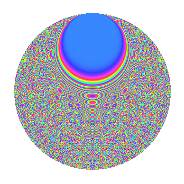# Properties

 Label 861.2.ciLevel 861 Weight 2 Character orbit ci Rep. character $$\chi_{861}(19,\cdot)$$ Character field $$\Q(\zeta_{120})$$ Dimension 1792 Newforms 1 Sturm bound 224 Trace bound 0

# Related objects

## Defining parameters

 Level: $$N$$ = $$861 = 3 \cdot 7 \cdot 41$$ Weight: $$k$$ = $$2$$ Character orbit: $$[\chi]$$ = 861.ci (of order $$120$$ and degree $$32$$) Character conductor: $$\operatorname{cond}(\chi)$$ = $$287$$ Character field: $$\Q(\zeta_{120})$$ Newforms: $$1$$ Sturm bound: $$224$$ Trace bound: $$0$$

## Dimensions

The following table gives the dimensions of various subspaces of $$M_{2}(861, [\chi])$$.

Total New Old
Modular forms 3712 1792 1920
Cusp forms 3456 1792 1664
Eisenstein series 256 0 256

## Trace form

 $$1792q + O(q^{10})$$ $$1792q + 8q^{14} - 224q^{16} + 48q^{19} - 80q^{22} - 72q^{24} - 48q^{26} + 64q^{29} - 16q^{30} - 40q^{32} - 72q^{37} - 72q^{38} + 64q^{42} - 64q^{43} - 192q^{44} - 24q^{46} + 48q^{47} - 160q^{49} + 128q^{50} + 72q^{52} - 16q^{53} + 112q^{56} + 8q^{58} - 64q^{65} + 32q^{67} - 744q^{68} + 160q^{70} + 32q^{71} - 72q^{73} + 56q^{74} - 384q^{75} - 32q^{77} + 96q^{78} - 8q^{79} + 72q^{80} - 576q^{82} + 240q^{84} + 32q^{85} - 88q^{88} - 384q^{89} + 112q^{91} + 216q^{94} + 64q^{95} - 480q^{96} - 40q^{98} - 32q^{99} + O(q^{100})$$

## Decomposition of $$S_{2}^{\mathrm{new}}(861, [\chi])$$ into irreducible Hecke orbits

Label Dim. $$A$$ Field CM Traces $q$-expansion
$$a_2$$ $$a_3$$ $$a_5$$ $$a_7$$
861.2.ci.a $$1792$$ $$6.875$$ None $$0$$ $$0$$ $$0$$ $$0$$

## Decomposition of $$S_{2}^{\mathrm{old}}(861, [\chi])$$ into lower level spaces

$$S_{2}^{\mathrm{old}}(861, [\chi]) \cong$$ $$S_{2}^{\mathrm{new}}(287, [\chi])$$$$^{\oplus 2}$$